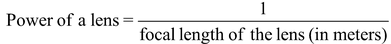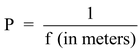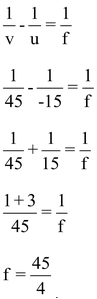# Power Of A Lens

## POWER OF A LENS

The power of a lens is the degree of convergence or divergence of light rays achieved by a lens.

The power of a lens is defined as the reciprocal of its focal length in meters. It is denoted by the letter P.

i.e.orwhere P = power of a lens

and f = focal length of the lens in meters.

The S.I. unit of power is dioptre. It is denoted by the letter ‘D’.

One dioptre is the power of a lens whose focal length is 1 metre.

The power of a lens is measured by an instrument called dioptre meter.

A convex lens has positive focal length so the power of a convex lens is positive.

A concave lens has a negative focal length, so the power of a concave lens is negative.

Since the power of a lens is inversely proportional to its focal length P = 1/f(in meters) Therefore, a lens of short focal length has more power than a lens of long focal length (i.e. shorter the focal length, more is the power and vice-versa).

### Power of a combination of lenses

If a number of lenses are placed in close contact, then the power of the combination of lenses is equal to the algebraic sum of the powers of individual lens.

i.e. P = P1 + P2 + P3 + ………

Where P = power of combination of lenses.

P1, P2, P3, ……………… = Powers of individual lens placed close to each other.

Ex. A convex lens produces an inverted image magnified three times of an object placed at a distance of 15 cm from it. Calculate focal length of the lens.

Solution: Here, magnification, m = –3

Object distance, u = –15 cm

And, image distance, v = ?

Now, for a lens, magnification,

m = v/u

-3 = v/-15

v = –3 × (–5)

Thus, image distance, v = +45 cm.

Also, for a lens:Thus, focal length, f = + 11.25 cm.